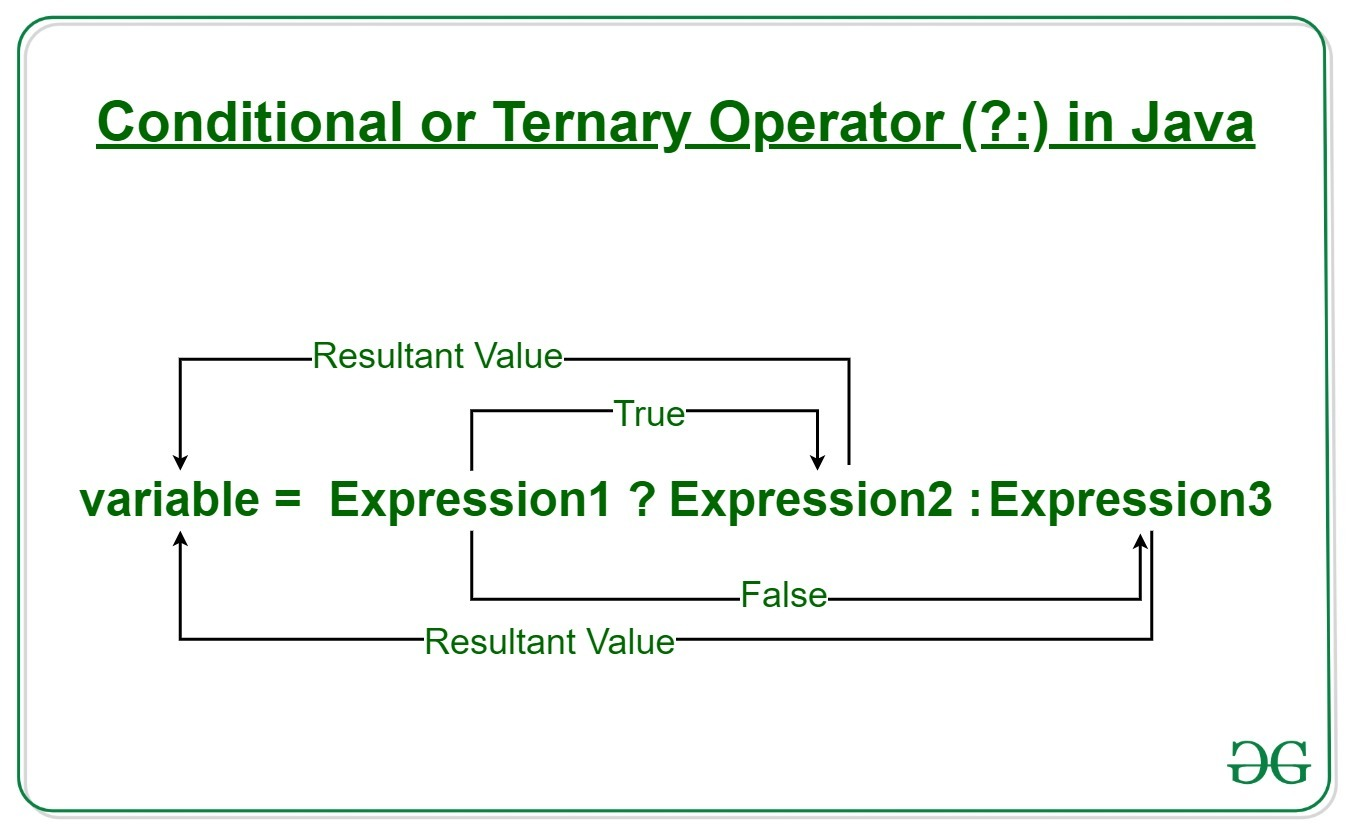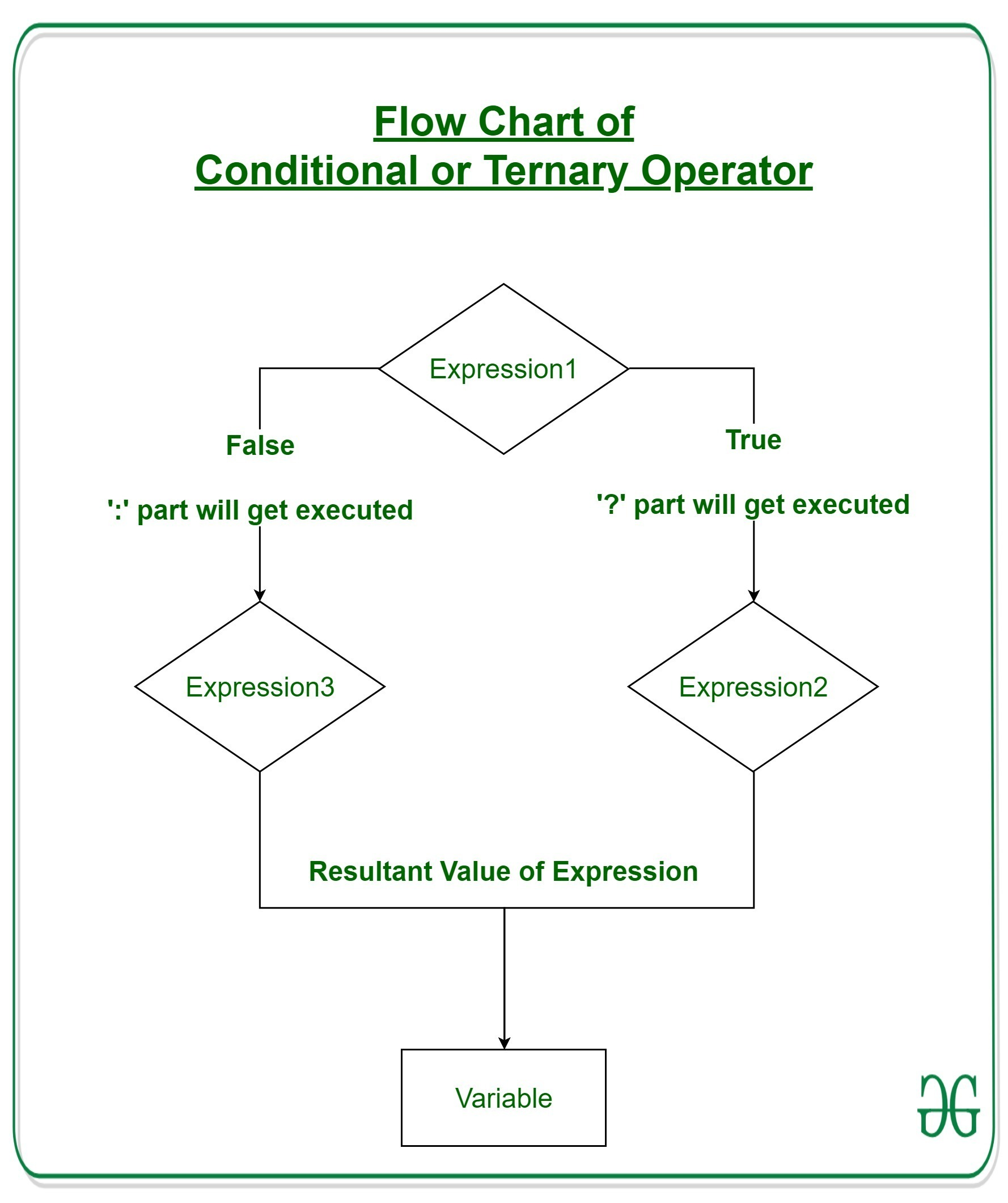Open in App
Not now

# Java Ternary Operator with Examples

• Difficulty Level : Easy
• Last Updated : 01 Dec, 2022

Operators constitute the basic building block to any programming language. Java too provides many types of operators which can be used according to the need to perform various calculations and functions, be it logical, arithmetic, relational, etc. They are classified based on the functionality they provide. Here are a few types:

This article explains all that one needs to know regarding Arithmetic Operators.

### Ternary Operator

Java ternary operator is the only conditional operator that takes three operands. It’s a one-liner replacement for the if-then-else statement and is used a lot in Java programming. We can use the ternary operator in place of if-else conditions or even switch conditions using nested ternary operators. Although it follows the same algorithm as of if-else statement, the conditional operator takes less space and helps to write the if-else statements in the shortest way possible.Syntax:

`variable = Expression1 ? Expression2: Expression3`

If operates similarly to that of the if-else statement as in Exression2 is executed if Expression1 is true else Expression3 is executed.

```if(Expression1)
{
variable = Expression2;
}
else
{
variable = Expression3;
}```

Example:

```num1 = 10;
num2 = 20;

res=(num1>num2) ? (num1+num2):(num1-num2)

Since num1<num2,
the second operation is performed
res = num1-num2 = -10```

#### Flowchart of Ternary OperationExample 1:

## Java

 `// Java program to find largest among two` `// numbers using ternary operator`   `import` `java.io.*;`   `class` `Ternary {` `    ``public` `static` `void` `main(String[] args)` `    ``{`   `        ``// variable declaration` `        ``int` `n1 = ``5``, n2 = ``10``, max;`   `        ``System.out.println(``"First num: "` `+ n1);` `        ``System.out.println(``"Second num: "` `+ n2);`   `        ``// Largest among n1 and n2` `        ``max = (n1 > n2) ? n1 : n2;`   `        ``// Print the largest number` `        ``System.out.println(``"Maximum is = "` `+ max);` `    ``}` `}`

Output

```First num: 5
Second num: 10
Maximum is = 10```

Time Complexity: O(1)
Auxiliary Space: O(1)

Example 2:

## Java

 `// Java code to illustrate ternary operator`   `import` `java.io.*;`   `class` `Ternary {` `    ``public` `static` `void` `main(String[] args)` `    ``{`   `        ``// variable declaration` `        ``int` `n1 = ``5``, n2 = ``10``, res;`   `        ``System.out.println(``"First num: "` `+ n1);` `        ``System.out.println(``"Second num: "` `+ n2);`   `        ``// Performing ternary operation` `        ``res = (n1 > n2) ? (n1 + n2) : (n1 - n2);`   `        ``// Print the largest number` `        ``System.out.println(``"Result = "` `+ res);` `    ``}` `}`

Output

```First num: 5
Second num: 10
Result = -5```

Time Complexity: O(1)
Auxiliary Space: O(1)

My Personal Notes arrow_drop_up
Related Articles